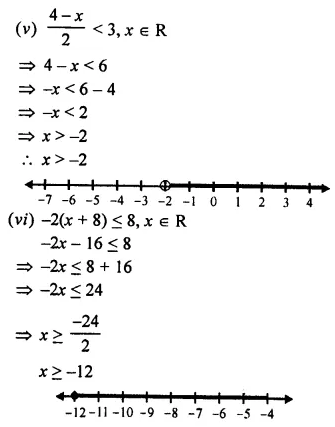## Selina Concise Mathematics Class 8 ICSE Solutions Chapter 15 Linear Inequations (Including Number Lines)

Selina Publishers Concise Mathematics Class 8 ICSE Solutions Chapter 15 Linear Inequations (Including Number Lines)

### Linear Inequations Exercise 15A – Selina Concise Mathematics Class 8 ICSE Solutions

Question 1.
If the replacement set is the set of natural numbers, solve.
(i) x – 5 < 0
(ii) x + 1 < 7
(iii) 3x – 4 > 6
(iv) 4x + 1 > 17
Solution:Question 2.
If the replacement set = {-6, -3, 0, 3, 6, 9}; find the truth set of the following:
(i) 2x – 1 > 9
(ii) 3x + 7 < 1
Solution: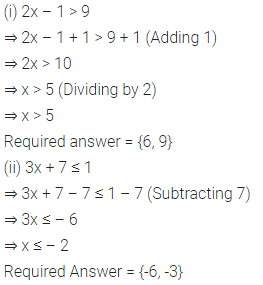Question 3.
Solve 7 > 3x – 8; x ∈ N
Solution:Question 4.
-17 < 9y – 8 ; y ∈ Z
Solution:Question 5.
Solve 9x – 7 ≤ 28 + 4x; x ∈ W
Solution: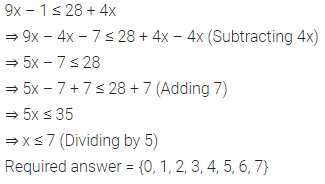Question 6.
Solve : $$\frac { 2 }{ 3 }$$x + 8 < 12 ; x ∈ W
Solution: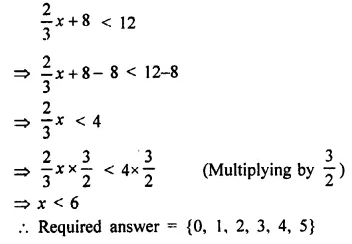Question 7.
Solve -5 (x + 4) > 30 ; x ∈ Z
Solution: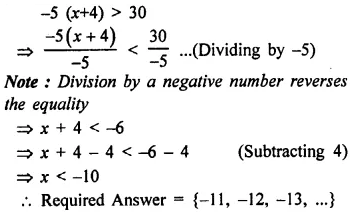Question 8.
Solve the inquation 8 – 2x > x – 5 ; x ∈ N.
Solution: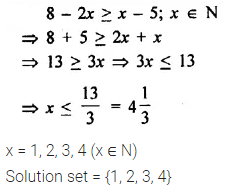Question 9.
Solve the inequality 18 – 3 (2x – 5) > 12; x ∈ W.
Solution: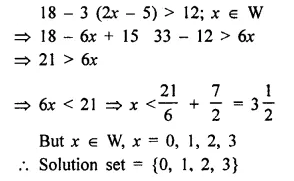Question 10.
Solve : $$\frac { 2x+1 }{ 3 }$$ + 15 < 17; x ∈ W.
Solution: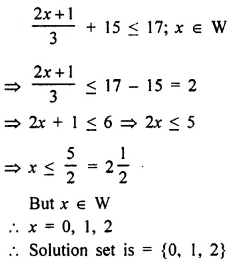Question 11.
Solve : -3 + x < 2, x ∈ N
Solution: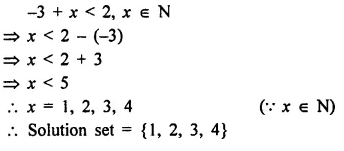Question 12.
Solve : 4x – 5 > 10 – x, x ∈ {0, 1, 2, 3, 4, 5, 6, 7}
Solution: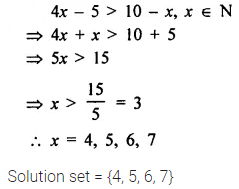Question 13.
Solve : 15 – 2(2x – 1) < 15, x ∈ Z.
Solution: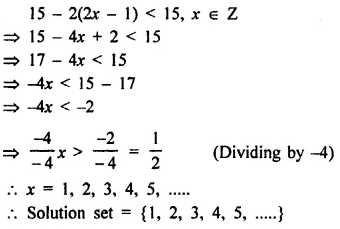Question 14.
Solve : $$\frac { 2x+3 }{ 5 }$$ > $$\frac { 4x-1 }{ 2 }$$ , x ∈ W.
Solution: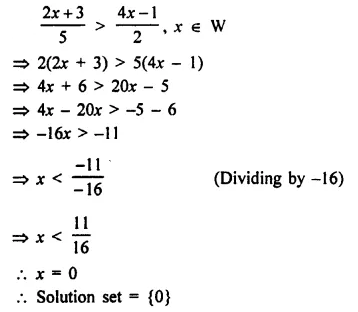### Linear Inequations Exercise 15B – Selina Concise Mathematics Class 8 ICSE Solutions

Solve and graph the solution set on a number line :
Question 1.
x – 5 < -2 ; x ∈ N
Solution: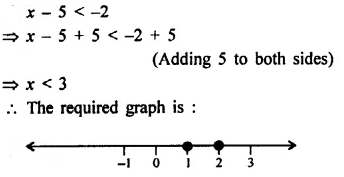Question 2.
3x – 1 > 5 ; x ∈ W
Solution: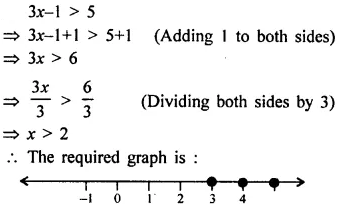Question 3.
-3x + 12 < -15 ; x ∈ R.
Solution: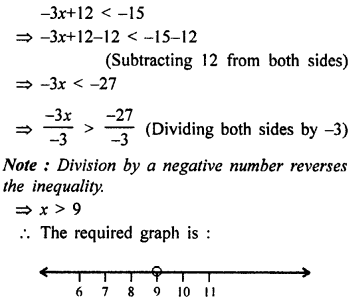Question 4.
7 > 3x – 8 ; x ∈ W
Solution: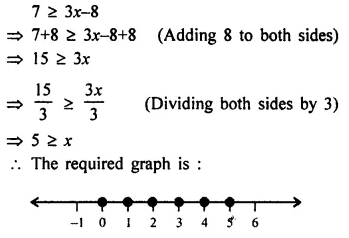Question 5.
8x – 8 < – 24 ; x ∈ Z
Solution: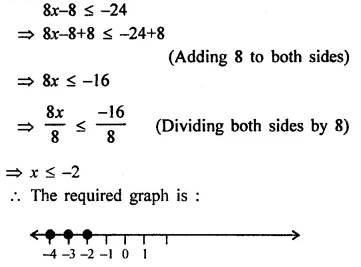Question 6.
8x – 9 > 35 – 3x ; x ∈ N
Solution: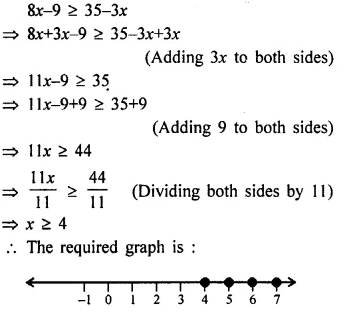Question 7.
5x + 4 > 8x – 11 ; x ∈ Z
Solution: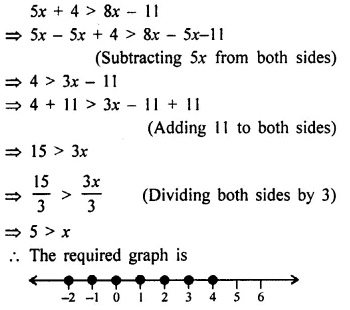Question 8.
$$\frac { 2x }{ 5 }$$ + 1 < -3 ; x ∈ R
Solution: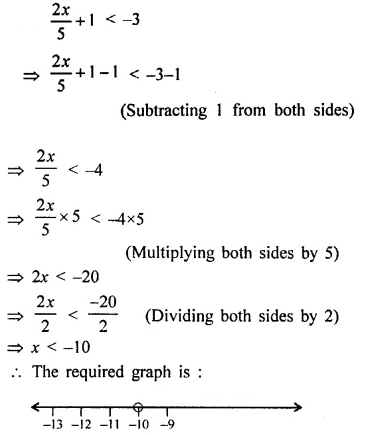Question 9.
$$\frac { x }{ 2 }$$ > -1 + $$\frac { 3x }{ 4 }$$ ; x ∈ N
Solution: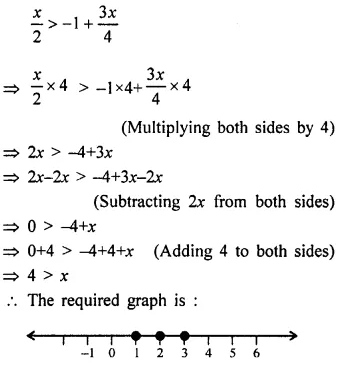Question 10.
$$\frac { 2 }{ 3 }$$ x + 5 ≤ $$\frac { 1 }{ 2 }$$ x + 6 ; x ∈ W
Solution: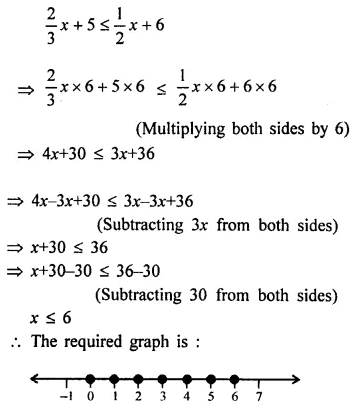Question 11.
Solve the inequation 5(x – 2) > 4 (x + 3) – 24 and represent its solution on a number line.
Given the replacement set is {-4, -3, -2, -1, 0, 1, 2, 3, 4}.
Solution:Question 12.
Solve $$\frac { 2 }{ 3 }$$ (x – 1) + 4 < 10 and represent its solution on a number line.
Given replacement set is {-8, -6, -4, 3, 6, 8, 12}.
Solution: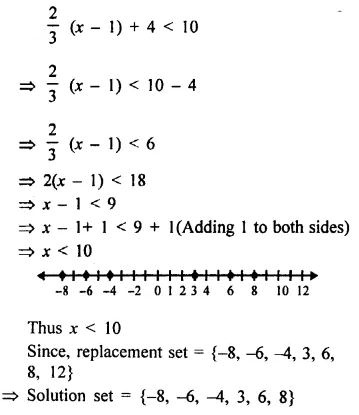Question 13.
For each inequation, given below, represent the solution on a number line :
(i) $$\frac { 5 }{ 2 }$$ – 2x ≥ $$\frac { 1 }{ 2 }$$ ; x ∈ W
(ii) 3(2x – 1) ≥ 2(2x + 3), x ∈ Z
(iii) 2(4 – 3x) ≤ 4(x – 5), x ∈ W
(iv) 4(3x + 1) > 2(4x – 1), x is a negative integer
(v) $$\frac { 4 – x }{ 2 }$$ < 3, x ∈ R
(vi) -2(x + 8) ≤ 8, x ∈ R
Solution: